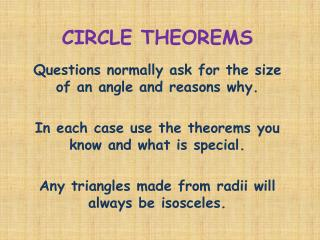Download PresentationCIRCLE THEOREMS

# CIRCLE THEOREMS - PowerPoint PPT PresentationDownload Presentation## CIRCLE THEOREMS

- - - - - - - - - - - - - - - - - - - - - - - - - - - E N D - - - - - - - - - - - - - - - - - - - - - - - - - - -
##### Presentation Transcript

1. CIRCLE THEOREMS Questions normally ask for the size of an angle and reasons why. In each case use the theorems you know and what is special. Any triangles made from radii will always be isosceles.

2. CIRCLE THEOREMS Tangent and Radius are perpendicular

3. CIRCLE THEOREMS Angle in a Semi-Circle is always 90°

4. CIRCLE THEOREMS The angle at the Centre is twice that at the circumference

5. CIRCLE THEOREMS Angles A and C are equal from the chord BD In the same way angle B and D are equal, coming from the chord AC Angles from the same chord are equal

6. CIRCLE THEOREMS A and C add to 180 and so do B and D The opposite angles in a cyclic quadrilateral add to 180°

7. CIRCLE THEOREMS Angle between the tangent and the chord (angle a on the diagram) equals the angle in the alternate(other) segment – angle b Alternate Segment theorem

8. Examples Try the following examples. The question is on one page, go to next page for a solution

9. CIRCLE THEOREMS Examples

10. In Δ OAB angles A and B are equal OA=OB as radii which makes isosceles Angle OBA will then be (180-2x) ÷ 2 which is (90-x) OB and OT are perpendicular as they are radius and tangent and so Angle ABT will be 90-(90-x) which is x CIRCLE THEOREMS Examples

11. CIRCLE THEOREMS Examples

12. Draw the line AO and label angles at B and C as b and c • In Δ OAC, • OA=OC as radii so Δ is isosceles • Angle OAC = OCA = c • Angle AOC is 180 – 2c • In same way in ΔOBC angle BOC is 180 – 2b • Angles around a point are 360 so angle BOC=360- AOC – BOC • = 360 – (180 -2b) –(180-2c) • = 2b + 2c • = 2 x ( b + c) = 2 x angle CAB CIRCLE THEOREMS b c Examples

13. The diagram shows a circle centre O. A, B and C are points on the circumference. DCO is a straight line. DA is a tangent to the circle. Angle ADO = 36° CIRCLE THEOREMS Examples (a) Work out the size of angle AOD° (b) Work out the size of angle ABC. Give reasons for your answers

14. Angle OAD is 90 as OA is a radius and AD a tangent Angle AOD is 54° as OAD is a triangle and OAD is 90.Angle ABC is the angle at the circumference when AOC is at the centre and so is 27° Angle at centre theorem CIRCLE THEOREMS

15. CIRCLE THEOREMS Examples

16. Angle PQT = angle PTA = 58° Alternate segment theorem In Δ PQT, PQ=QT and so it isosceles and the angles at P and T are equal and as PQT is 58, they are (180-58) ÷ 2 which is 61 Angle POT is 90-58 = 32 because angle OAT is 90 (radius/tangent) Finally OTQ = 61-32 = 29° CIRCLE THEOREMS

17. CIRCLE THEOREMS Examples

18. AD is a chord and angles ABD and ACD are from that chord and so are equal.  ACD = 54° CIRCLE THEOREMS

19. CIRCLE THEOREMS Examples

20. ABCD is a cyclic quadrilateral and so angle BAD = 50 Angle BOD is the angle at the centre and so is 100° In the quadrilateral OBDC we now have 100 and 130 which leaves 360-230 = 130 for the other two. As OB=OD (radii), OBDC is symmetric and angle OBC and ODC are equal and must be 130 ÷ 2 = 65° CIRCLE THEOREMS

21. CIRCLE THEOREMS Examples

22. ABCD is a cyclic quadrilateral and so angle D is 52° Angle AOC is the angle at the centre and so will be twice that at the circumference and so is 2 x 52 = 104° CIRCLE THEOREMS

23. CIRCLE THEOREMS Examples

24. As no angles are known set the one you want to x BC=BD so Δ BCD is isosceles and so BCD is also x Angle C is 90 as it is the angle in a semi-circle and so angle ACD is 90 - x Because AC and BD are parallel, angles ACD and CDB are equal (alternate angles) and so x = 90 – x Solving gives x = 45° CIRCLE THEOREMS